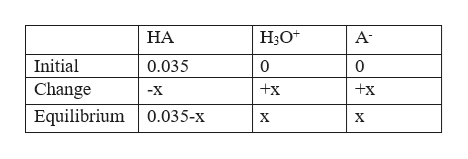# Calculate the pH at 25°C of a 0.035 M solution of a weak acid that has Ka = 1.7×10−5.

Question
1 views

Calculate the pH at 25

°

C of a 0.035 M solution of a weak acid that has Ka = 1.7

×

10−5.

check_circle

Step 1

Given:

Ka = 1.7×10−5.

Weak acid [HA] = 0.035 M.

Step 2

Chemical reaction is given by:

Step 3

Equilibrium concentrati...help_outlineImage TranscriptioncloseH3O* НА A- Initial 0.035 0 0 Change +x +x -X Equilibrium 0.035-x X X fullscreen

### Want to see the full answer?

See Solution

#### Want to see this answer and more?

Solutions are written by subject experts who are available 24/7. Questions are typically answered within 1 hour.*

See Solution
*Response times may vary by subject and question.
Tagged in

### Chemistry